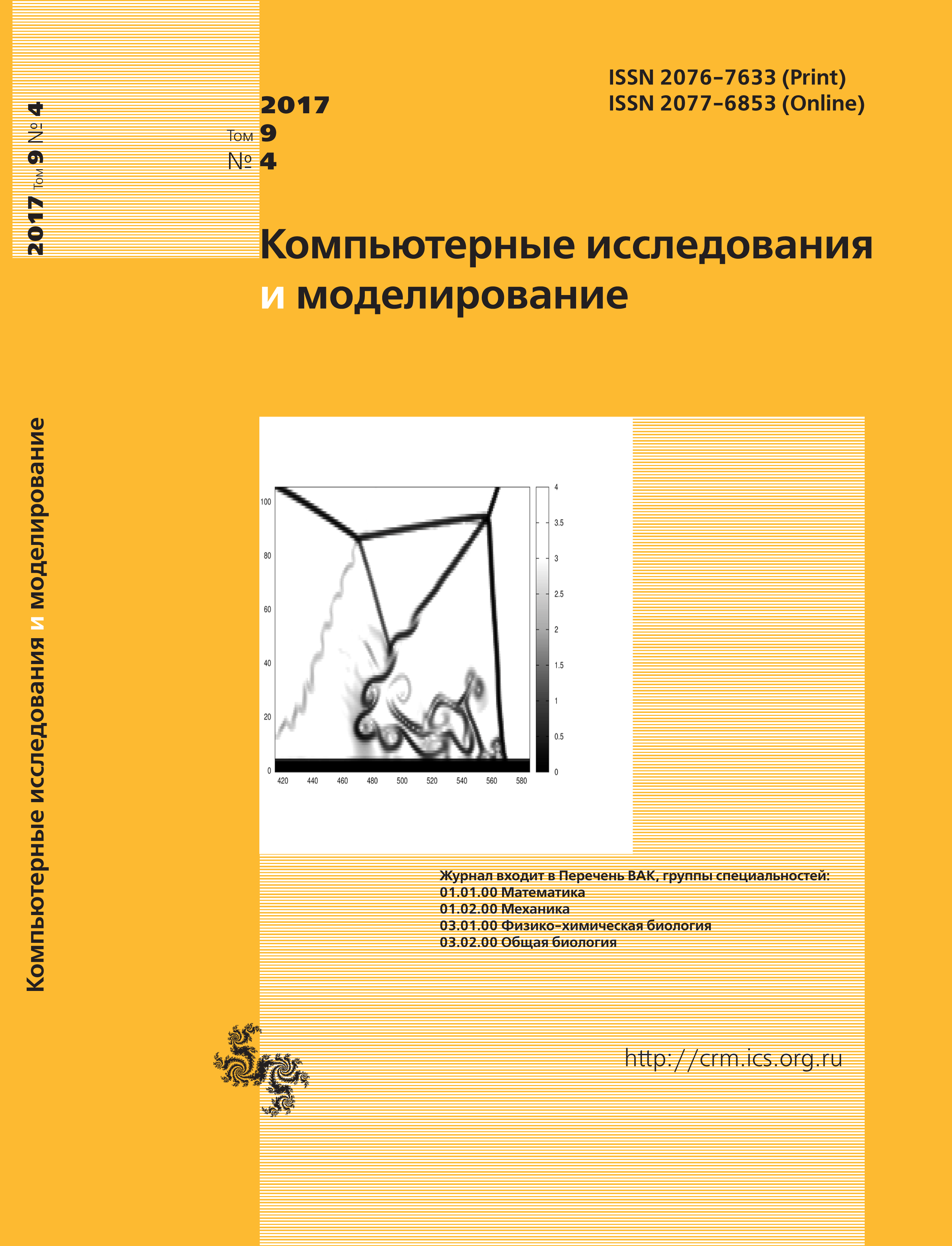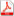Issue 4, 2017 Vol. 9

# All issues

Cellular automata methods in mathematical physics classical problems solving on hexagonal grid. Part 2pdf (5342K)  / List of references

The second part of paper is devoted to final study of three classic partial differential equations (Laplace, Diffusion and Wave) solution using simple numerical methods in terms of Cellular Automata. Specificity of this solution has been shown by different examples, which are related to the hexagonal grid. Also the next statements that are mentioned in the first part have been proved: the matter conservation law and the offensive effect of excessive hexagonal symmetry.

From the point of CA view diffusion equation is the most important. While solving of diffusion equation at the infinite time interval we can find solution of boundary value problem of Laplace equation and if we introduce vector-variable we will solve wave equation (at least, for scalar). The critical requirement for the sampling of the boundary conditions for CA-cells has been shown during the solving of problem of circular membrane vibrations with Neumann boundary conditions. CA-calculations using the simple scheme and Margolus rotary-block mechanism were compared for the quasione-dimensional problem “diffusion in the half-space”. During the solving of mixed task of circular membrane vibration with the fixed ends in a classical case it has been shown that the simultaneous application of the Crank–Nicholson method and taking into account of the second-order terms is allowed to avoid the effect of excessive hexagonal symmetry that was studied for a simple scheme.

By the example of the centrally symmetric Neumann problem a new method of spatial derivatives introducing into the postfix CA procedure, which is reflecting the time derivatives (on the base of the continuity equation) was demonstrated. The value of the constant that is related to these derivatives has been empirically found in the case of central symmetry. The low rate of convergence and accuracy that limited within the boundaries of the sample, in contrary to the formal precision of the method (4-th order), prevents the using of the CAmethods for such problems. We recommend using multigrid method. During the solving of the quasi-diffusion equations (two-dimensional CA) it was showing that the rotary-block mechanism of CA (Margolus mechanism) is more effective than simple CA.

Keywords: cellular automata with continuous values, hexagonal grid, finite-difference methods, partial differential equations, PDEs
Citation in English: Matyushkin I.V. Cellular automata methods in mathematical physics classical problems solving on hexagonal grid. Part 2 // Computer Research and Modeling, 2017, vol. 9, no. 4, pp. 547-566
Citation in English: Matyushkin I.V. Cellular automata methods in mathematical physics classical problems solving on hexagonal grid. Part 2 // Computer Research and Modeling, 2017, vol. 9, no. 4, pp. 547-566
DOI: 10.20537/2076-7633-2017-9-4-547-566
Views (last year): 6.

Full-text version of the journal is also available on the web site of the scientific electronic library eLIBRARY.RU

The journal is included in the Russian Science Citation Index

The journal is included in the List of Russian peer-reviewed journals publishing the main research results of PhD and doctoral dissertations.

International Interdisciplinary Conference "Mathematics. Computing. Education"

The journal is included in the RSCI

Indexed in Scopus